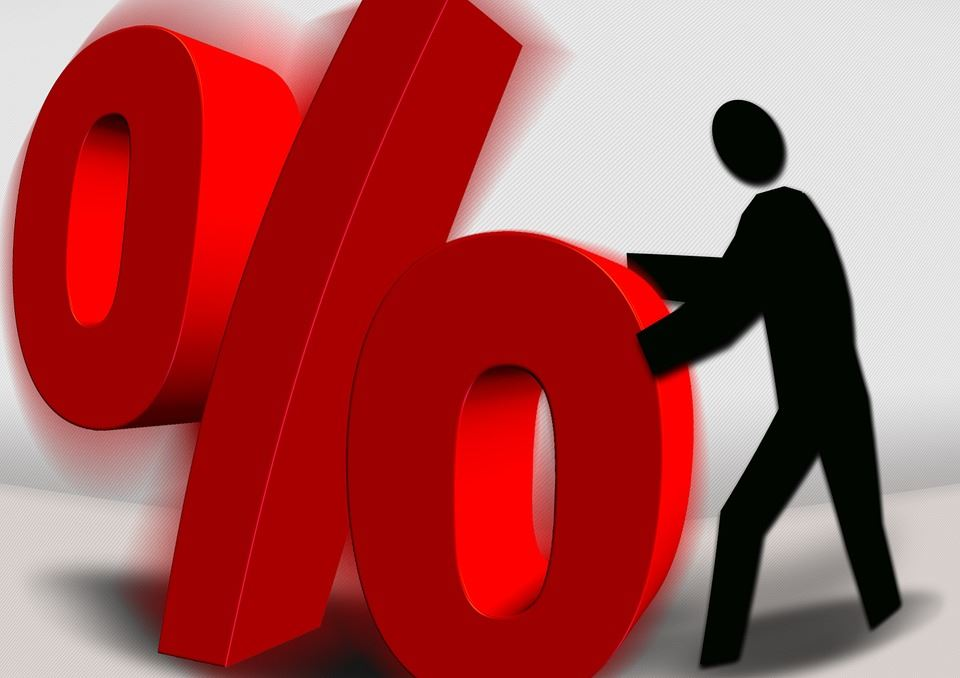Percentages: Interpreting And Converting Percentages

Before talking about ‘interpreting and converting percentages’, let us quickly recall what percentage is. We have often seen percentage as a way to evaluate a student’s performance in examinations. It simplifies the task of comparing two students’ results. Percentage is, thus, a way to compare quantities. It literally means ‘per 100’, which is a number expressed as a fraction of 100. So when you say 100% of something, it means it represents the entire quantity. That being said, in this article, we will discuss how percentage is interpreted and about converting percentages to ‘how many’.Some of the uses of percentage are:

• Interpreting and converting percentages
• Ratio to percentage
• Increase or decrease as percentage

Interpreting Percentages

Percentage is useful in comparison. Any two quantities, when represented in their percentage form, can easily be assessed. This is because no matter what the numbers are, when they are converted to the form of ‘per 100’, their interpretation is simplified. Consider the following statements:

• Mita scored 89% in the Math test.
• Saurav spent 30% of his pocket money.
• 10% of Anita’s candies are strawberry.

They can be interpreted in the following way:

• Mita scored 89 out of 100 marks.
• Saurav spends Rs 30 for every Rs 100 pocket money that he gets.
• Out of every 100 candies that Anita has, 10 of them are strawberry.

This is how we interpret quantities with the help of percentage in real life.

Converting Percentages

We know that percentage is a quantity expressed ‘out of 100’. So when we say that 10% of Anita’s candies are strawberry, it does not necessarily mean that Anita has 100 candies. It may be possible that Anita has 50 candies, out of which 10% are strawberry. Thus, one of the uses of percentage is converting percentages to ‘how many’. Let us take the same example to understand this.

Question: Anita has 50 candies out of which 10% are strawberry. Find the number of strawberry candies that she has.

Solution: This can be solved using either of the following two methods:

1. Out of 100 candies, 10 are strawberry

So out of 1 candy,  10/100 is strawberry

So out of 50 candies, the number of strawberry candies are  10/100 x 50 = 5

1. 10% of 50 candies are strawberry

10/100 x 50= 5 candies are strawberry

Now consider a simple example for converting percents into fraction.

Question: Convert 75% to a fraction

Solution: $\frac{75}{100}=\frac{15}{20}=\frac{3}{4}$<